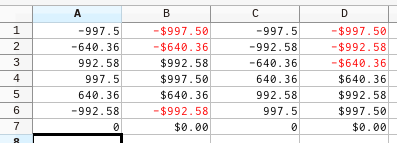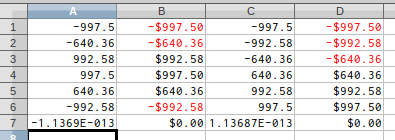# Calc - Sum error for currency

4.2.1.1 English Calc. Windows 8.1 English

If I sum a column of money, both credits and debits, I get a fraction of a cent error. When the debits and credits are next to each other, the sum correctly calculates 0.
sum these values: -997.5, -640.36, 992.58, 997.5, 640.36, -992.58 = -1.1369E-013
sum the values in order: -997.5, 997.5, -640.36, 640.36, 992.58, -992.58 = 0

It makes no difference if the cells are formatted for currency. Is this a bug, or am I doing something wrong?

• Gary

See this question.

There does appear to be a problem change in value handling in the v4.2.x series with respect to displayed precision. Given the basic test ODS here, created in v4.1.4.2, I am seeing this in v4.1:… and this in v4.2, v4.3, and the daily build (deb x86_64 2014-08-26) of v4.4:Sorting does not seem to make any difference to the result, but cell format does. I cannot locate an existing open bug about this so it may in fact be a bug, although I will let others confirm that I have not overlooked something. If someone does report a bug please feel free to use the example file and screenshots provided. As pointed out in the answer by @erAck, the IEEE 754 Double (64bit) precision has limitations for raw calculations that can result in rounding errors of this nature. Thanks @erAck for reminding me about this - I was thinking the error magnitude too small, but of course with greater precision (64bit) the error will be come smaller, rather than larger. Sigh.

Confirmed the issue, also in Master.

That’s normal because the currency format rounds the displayed value to 2 decimals. Calculations do not always result in exact numbers, there may be round-off errors because not all decimal numbers can be exactly represented as binary floating point values.

If you want to exactly calculate with currency amounts, either apply the correct rounding to all formula results using the ROUND(value,2) function, or format all value and formula cells with the correct number of decimals (e.g. as currency) and switch on Tools → Options → Calc → Calculate “Precision as shown”.

@erAck, given all the entered values contain only two decimal places, if the difference was a single 1/100th of the currency value, then I could understand this being a rounding error, but `-1.1369E-013` is a tiny value that indicates improper storage of a two-decimal place value somewhere e.g., `-997.50000000000011369` instead of `-997.5`. For an entered value this should not be happening, whether values are rounded or not. It seems a regression, given v4.1 does not exhibit this issue.

You may want to get familiar with the IEEE 754 floating point representation and read Numeric precision in Microsoft Excel - Wikipedia and https://support.microsoft.com/kb/78113

@erAck, you have my utter respect, so I am sorry if my query did not come across sounding like it. I was aware of IEEE 754 (similar query here) so should have known better, but thanks for the links in any case. I can see that 32bit signed binary representation of +/-640.36 and +/-992.58 is impossible (result in decimal is +/-640.35999989509582519531 and +/-992.5799999237060546875). I guess I am just having a memory lapse.

Objection: this is not at all normal. Decimal values like currencies should not be stored as binary floating point approximations. I observe a similar behaviour in 5.4.5, but it contradicts the documentation: “internally, the number retains all four decimal places after the decimal point”.

Perfect! That was exactly what I was looking for! Thank you!